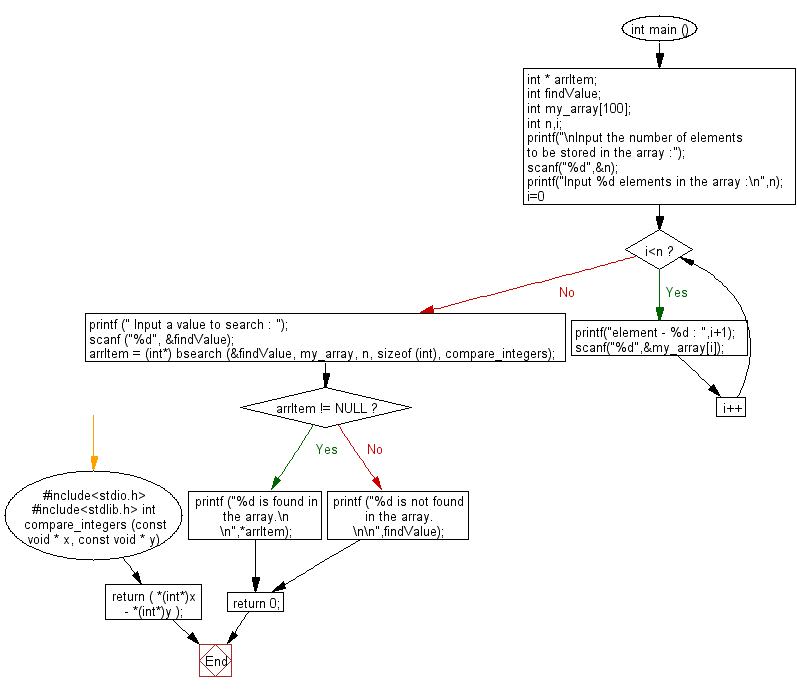﻿ C Program: Perform a binary search in an array - w3resource

# C Exercises: Perform a binary search in an array

## C Variable Type : Exercise-12 with Solution

Write a C program to perform a binary search in an array.

Sample Solution:

C Code:

``````#include<stdio.h>      // Include the standard input/output header file.
#include<stdlib.h>     // Include the standard library header file.

// Comparator function used for bsearch.
int compare_integers (const void * x, const void * y)
{
return ( *(int*)x - *(int*)y );
}

int main ()          // Start of the main function.
{
int * arrItem;    // Declare a pointer to int 'arrItem'.
int findValue;    // Declare an integer variable 'findValue'.
int my_array;   // Declare an integer array 'my_array' with a maximum size of 100.
int n, i;         // Declare integer variables 'n' and 'i'.

printf("\nInput the number of elements to be stored in the array :");   // Prompt the user to input the number of elements.
scanf("%d",&n);   // Read the user's input and store it in 'n'.

printf("Input %d elements in the array :\n",n);   // Prompt the user to input the elements of the array.
for(i=0;i<n;i++)   // Start of a for loop to iterate over the elements of the array.
{
printf("element - %d : ",i+1);   // Prompt the user for the value of the current element.
scanf("%d",&my_array[i]);     // Read the user's input and store it in the current element of 'my_array'.
}

printf (" Input a value to search : ");   // Prompt the user to input a value to search for.
scanf ("%d", &findValue);   // Read the user's input and store it in 'findValue'.

arrItem = (int*) bsearch (&findValue, my_array, n, sizeof (int), compare_integers);   // Search for 'findValue' in 'my_array'.

if (arrItem != NULL)   // If 'arrItem' is not NULL (i.e., if 'findValue' was found in the array).
printf ("%d is found in the array.\n\n",*arrItem);   // Print a message indicating that 'findValue' was found.
else

return 0;   // Return 0 to indicate successful execution of the program.
}   // End of the main function.
```
```

Sample Output:

```Input the number of elements to be stored in the array :5
Input 5 elements in the array :
element - 1 : 10
element - 2 : 15
element - 3 : 20
element - 4 : 25
element - 5 : 30
Input a value to search : 20
20 is found in the array.
```

Flowchart:C Programming Code Editor:

What is the difficulty level of this exercise?

Test your Programming skills with w3resource's quiz.

﻿